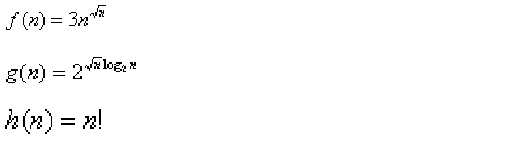Related Articles
Algorithms | Analysis of Algorithms | Question 15
• Last Updated : 20 Feb, 2013

Consider the following functionsWhich of the following is true? (GATE CS 2000)
(a) h(n) is 0(f(n))
(b) h(n) is 0(g(n))
(c) g(n) is not 0(f(n))
(d) f(n) is 0(g(n))
(A) a
(B) b
(C) c
(D) d

Explanation: g(n) = 2^= n^(a) and (b) are false because n! is of asymptotically higher order than n^.